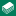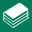# Course Information

Basic Mathematics: Formulas and Operations
 Release Date: January 17, 2018Delivery: OnlinePresentation Style: DocumentaryContinuing Education Qualification: NoneApproximate Time: 1.5 hours Synopsis: This course is designed to provide the basic knowledge needed when solving mathematical equations. It is important to have a solid understanding of math when working with the ASME Code.GeneralEnroll Now! - \$100.00General Information

Basic Mathematics: Operations and Formulas, is an online coursedesigned to provide the basic knowledge needed when solving mathematical equations. It is important to have a solid understanding of math when working with the ASME Code.

Throughout this course, students will gain an understanding for order of operations, working with formulas, rounding numbers, and Pi. Students will also learn how to solve for area, perimeter, circumference, lengths and span. Practice problems are offered throughout the course along with several practice applications in the final module.

Working straight through the material, it is estimated the course takes approximately four to five hours to complete.

Learning Outcomes
After completing this course, students will be able to:

• Solve mathematical equations using the order of operations.
• Describe the steps for rounding numbers to designated place values.
• Define basic shapes based on number of sides and angles.
• Calculate the following: area, perimeter, circumference, side lengths, and short span.
• Identify and use appropriate formulas to solve for unknown information (variables).

Reference Materials
Each student is required to have a calculator, pencil, and paper.

Criteria for Successful Completion
As a minimum, for a National Board Certificate of Completion to be issued, students must:

• actively participate in all online course interactions
• respond to all knowledge check questions